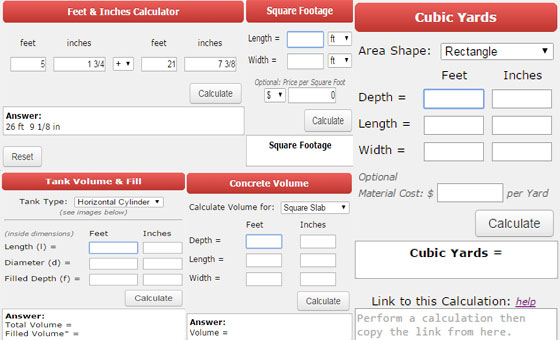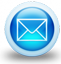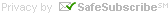# Types of online construction calculators and their usages

## Home » Construction take-off sheets » Earthwork Volume Calculation Sheet

The online construction calculators facilitate the building contractor to make cost estimation instantly in the jobsite for different types of construction materials associated with a construction project. This calculator can help you to make accurate estimated cost of any building. One can also search out thorough and instant cost breakups with multiple options.

Feet and Inches Calculator: This calculator is specifically designed to add, subtract, multiply and divide feet and inches fractions. The calculator deals with functioning on feet whole numbers and inches in mixed numbers to add, subtract, divide or multiply whole numbers, fractions as well as mixed numbers and present the result in an abridged fraction and a mixed number, if it prevails.

Put in inches in mixed numbers, whole numbers or fractions in the formats given below:

• Mixed Numbers accurately as 1 1/2 describing one and one half or 25 3/32 refers to twenty five and three thirty seconds. Keep precisely one space amid the whole number and fraction.
• Whole numbers up to 3 digits in length. (123)
• Fractions as 3/4 refers to three fourths or 3/100 describing three one hundredreths.

Concrete Volume Calculator: This calculator is useful to compute volumes for concrete slabs, walls, footers and columns. Put in dimensions for the concrete structure in measurement unit of feet and inches to obtain thu cubic yards value of the amount of concrete required to form this structure.

Cubic Yards Calculator: Construction professionals use this calculator to estimate cubic yards for various construction elements like landscape material, land fill, gravel or containers. In order to utilize this calculator, just provide the width, length, and depth of the area to be filled up. Afterward, opt for either inches or feet used for the depth and press the calculate button to obtain the cubic yards value of the required material amount.

Square Footage Calculator: While constructing a house, setting up flooring, tile or painting a house, this calculator helps you to calculate the square footage to estimate the required amount of material or cost.

This calculator is well suited with different shapes like rectangle, triangle, trapezoid, or circle.

The measurements for calculations may vary from inches (in), feet (ft), yards (yd), centimeters (cm), or meters (m).

The most convenient way for square footage calculation is to work it out by measuring the length and width of the area in feet and multiplying those two numbers collectively to obtain the area in feet squared. This is useful for a rectangular area like a room as well as calculating square footage for flooring or carpet. While making calculation in other units except feet, initially transfer those values to feet, then multiply them collectively to obtain the square footage of the area.

Roadway Fill Volume Calculator: This calculator is used to work out roadway as well as parking lot material fill essential for construction or maintenance projects. This calculator facilitates the user to provide common road materials or custom entry so that they can put their own material density.

Units of measure are arranged for being applied all over the world and prices are computed for total per ton of material.

For making calculations with irregular shapes, just break them down into a series of regular shapes. After that, combine the parts.

Tank Volume & Fill Calculator: This calculator is utilized to calculate the total and filled volumes of tanks like oil tanks and water tanks.

Guesses inside dimensions of the tank.

Tank volume calculations are made on the basis of tank geometries shown given below. These tank shapes are measured presuming proper geometric solid shapes like cylinders, circles and spheres. Actual water and oil tanks dont have always exact geometric shapes or there are other features not accounted for here so, these calculations must be considered estimations.

These calculations will provide you cubic measures like ft3 or m3 conditional on your units of measure.Ref: www.calculatorsoup.com

# Takeoff Tools

DISCLAIMER: "Autodesk, AutoCAD, Revit, Navisworks, Ecotect, QTO, DWG, 3ds Max, are registered trademarks or trademarks of Autodesk, Inc. All other brand names, product names, or trademarks belong to their respective holders. © 2010 Autodesk, Inc. All rights reserved.

Site Design & Develop by